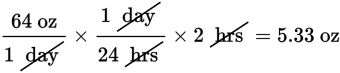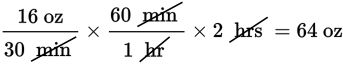# SAT Math Multiple Choice Question 472: Answer and Explanation

### Test Information

Question: 472

7. There are many ways to defrost a turkey. One way is to let it thaw slowly in the refrigerator, at a thaw rate of about 4 pounds per day. Another way is to submerge the turkey in cold water, which thaws it at a rate of 1 pound per 30 minutes. Approximately how many more ounces of turkey can the cold-water method thaw in 2 hours than the refrigerator method? (1 pound = 16 ounces)

• A. 16
• B. 27
• C. 32
• D. 59

Explanation:

D

Difficulty: Medium

Category: Problem Solving and Data Analysis / Rates, Ratios, Proportions, and Percentages

Strategic Advice: Let the units in this question guide you to the solution. The thawing rates of the different methods are given in pounds, but one is given in pounds per day and the other in pounds per 30 minutes. The question asks about the number of ounces each method can thaw in 2 hours, so convert pounds per day and pounds per 30 minutes to ounces per hour.

Getting to the Answer: Start by converting pounds to ounces. There are 16 ounces in 1 pound, so 4 pounds is 64 ounces and 1 pound is 16 ounces. Now, use the factor-label method to incorporate the time conversions.

Refrigerator Method:Cold Water Method:In 2 hours, the cold water method can thaw approximately 64 – 5.33 = 58.67 or about 59 more ounces.